# Aggressive Cows : Detailed Solution

Problem Statement: There is a new barn with N stalls and C cows. The stalls are located on a straight line at positions x1,….,xN (0 <= xi <= 1,000,000,000). We want to assign the cows to the stalls, such that the minimum distance between any two of them is as large as possible. What is the largest minimum distance?

Examples:

```Input: No of stalls = 5
Array: {1,2,8,4,9}
And number of cows: 3

Output: One integer, the largest minimum distance 3

```

Explanation: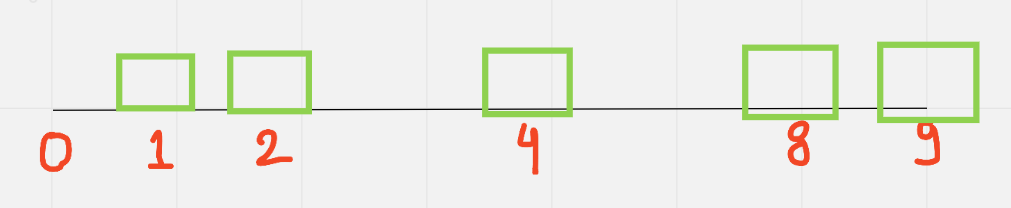We have to fit in three cows in these 5 stalls. Each stall can accommodate only one. Our task is to maximize the minimum distance between two stalls. Let’s look at some arrangements: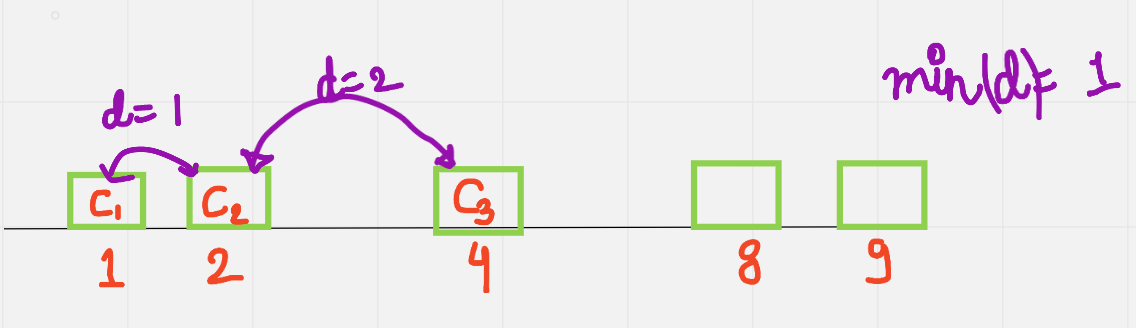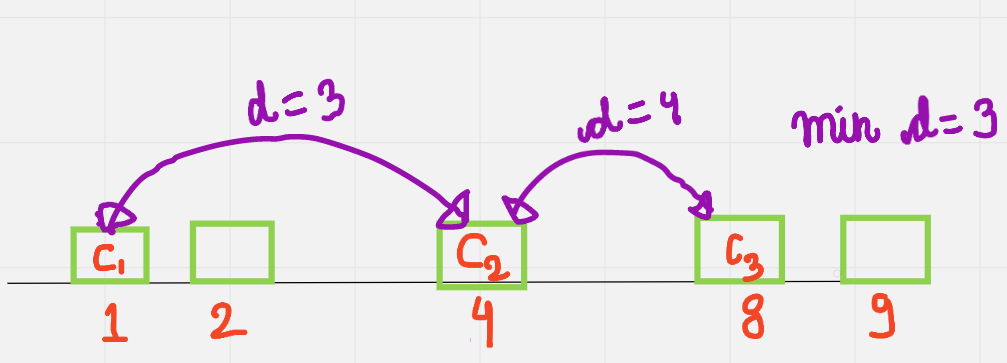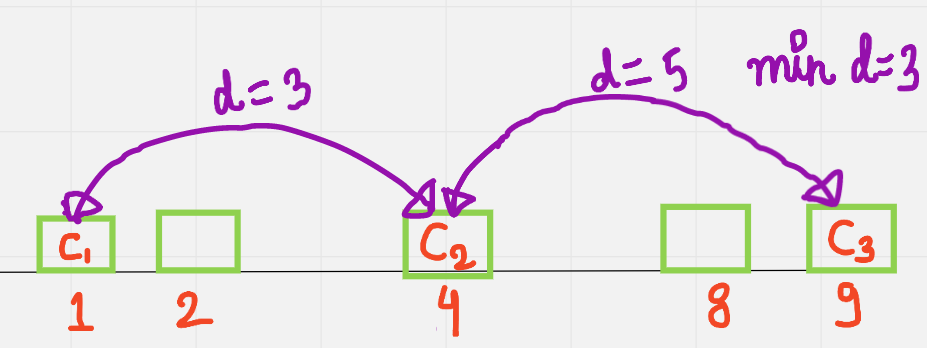In the first case, the cows were arranged in the first three consecutive stalls, which is not advisable because they require maximum distance between them. So we make sure that there is some minimum distance between them so they do not fight. We have to maximize that difference so as to accommodate three cows. This is done in the second and third examples. It’s not possible to get a minimum distance of more than 3 in any arrangement, so we output 3.

### Solution

Disclaimer: Don’t jump directly to the solution, try it out yourself first.

Solution 1: Brute Force

Intuition:

It’s required that we put all the C cows into our stalls. So for a start, let’s say we set the minimum distance = 1 and put them consecutively. These cows fit perfectly.

This is too close, so let’s increase our minimum distance a bit. Let’s increase the distance further to 2. We can again check that the cows can be accommodated.

But we want to reduce the possibility of collision/fighting as much as possible, so we keep on increasing the minimum distance. Here is an illustration: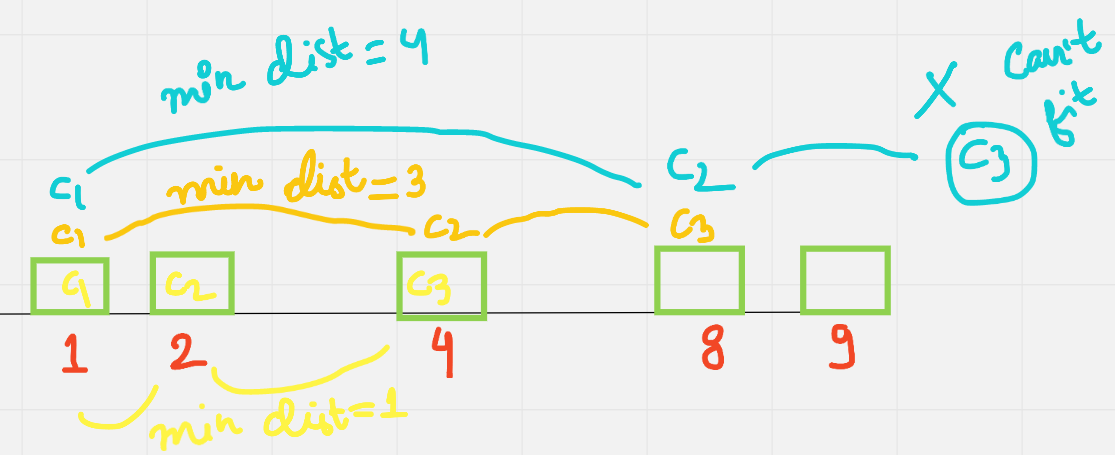Approach:

After sorting the array, we set a minimum distance, then we keep on increasing until accommodation of all cows is not possible. We stop just before that to get our answer, which in this example is 3.

For checking if the cows can fit or not, we are simply iterating over our n stalls, and for every stall with the said minimum distance, we place our cow. After we are done, if all cows have been accommodated, we return true, otherwise false. Let’s observe the time complexity of our brute force algorithm here, we are increasing distance in each step (which in the worst case of two cows gets as high as m = array[n-1]-array), and in that step, we are checking if our cows can “fit”, so we are iterating again for each step to check.

Code:

## C++ Code

``````#include <bits/stdc++.h>

using namespace std;
bool isCompatible(vector < int > inp, int dist, int cows) {
int n = inp.size();
int k = inp;
cows--;
for (int i = 1; i < n; i++) {
if (inp[i] - k >= dist) {
cows--;
if (!cows) {
return true;
}
k = inp[i];
}
}
return false;
}
int main() {
int n = 5, m = 3;
vector<int> inp {1,2,8,4,9};
sort(inp.begin(), inp.end());
int maxD = inp[n - 1] - inp;
int ans = INT_MIN;
for (int d = 1; d <= maxD; d++) {
bool possible = isCompatible(inp, d, m);
if (possible) {
ans = max(ans, d);
}
}
cout << "The largest minimum distance is " << ans << '\n';
}``````

Output: The largest minimum distance is 3

Time complexity: O(n* m)

Space Complexity: O(1)

## Java Code

``````import java.util.*;
class TUF {
static boolean isCompatible(int inp[], int dist, int cows) {
int n = inp.length;
int k = inp;
cows--;
for (int i = 1; i < n; i++) {
if (inp[i] - k >= dist) {
cows--;
if (cows == 0) {
return true;
}
k = inp[i];
}
}
return false;
}
public static void main(String args[]) {
int n = 5, m = 3;
int inp[] = {1,2,8,4,9};
Arrays.sort(inp);
int maxD = inp[n - 1] - inp;
int ans = Integer.MIN_VALUE;
for (int d = 1; d <= maxD; d++) {
boolean possible = isCompatible(inp, d, m);
if (possible) {
ans = Math.max(ans, d);
}
}
System.out.println("The largest minimum distance is " + ans);
}
}``````

Output: The largest minimum distance is 3

Time complexity: O(n* m)

Space Complexity: O(1)

Solution 2: Binary Search

Intuition:

Observing what we did in the previous case, this time complexity can be improved.

There is a certain maximum “limit” as to what we can increase our minimum distance because we don’t want any cow to be left behind.In this example, all values including and after 4 are invalid. There is certain “monotonicity” here, speaking in layman’s terms, we are trying to find d for which the minimum distance is maximized, and there is a certain value, here 4 including and after which the solution to this problem is not possible. In these situations, we use the binary search algorithm.

Approach:

(Note, before proceeding, make sure you are familiar with binary search.)

Make sure to sort the array first, because only then it makes sense to use binary search.

For the BS approach, we set low = 1 because the minimum distance is 1 and high =array[n-1] – array . because that’s the maximum possible distance between two stalls.

Let’s calculate our mid-value after this.

mid = low + (high-low)/2

Then we check if the minimum distance(mid-value) is possible by the same method defined in brute force, and if it is not possible, this means we can set our upper bound as high-1, and if it is possible, we store it in an answer variable and set our lower bound as mid+1. We keep on doing this until high and low pointers are equal.

Dry run of this example:

low = 1, high = 9-1 = 8

mid  = 1 + (8-1)/2 = 4

Let’s check for 4, but it doesn’t fit( check Fig 4), so now we can reduce our search space by setting the upper bound as 4-1=3 because all numbers greater than equal to 4 are not valid. Now we check for mid = 1 + (3-1)/2 = 2Which is 2, checking if it’s a valid solution and cows can fit in, we find that it is a valid solution, so we store it as a possible answer and because we need a maximum-minimum distance, we set the lower bound as 2+1 =3. We find that 3 is also a possible solution, so we store it in our ans because it’s greater than our previous answer. Our low and high variables are equal now, so we can stop our binary search here.

Code:

## C++ Code

``````    #include <bits/stdc++.h>

using namespace std;
bool isPossible(int a[], int n, int cows, int minDist) {
int cntCows = 1;
int lastPlacedCow = a;
for (int i = 1; i < n; i++) {
if (a[i] - lastPlacedCow >= minDist) {
cntCows++;
lastPlacedCow = a[i];
}
}
if (cntCows >= cows) return true;
return false;
}
int main() {
int n = 5, cows = 3;
int a[]={1,2,8,4,9};
sort(a, a + n);

int low = 1, high = a[n - 1] - a;

while (low <= high) {
int mid = (low + high) >> 1;

if (isPossible(a, n, cows, mid)) {
low = mid + 1;
} else {
high = mid - 1;
}
}
cout << "The largest minimum distance is " << high << endl;

return 0;
}``````

Output: The largest minimum distance is 3

Time Complexity: O(N*log(M)).

Reason: For binary search in a space of M, O(log(M))  and for each search, we iterate over max N stalls to check. O(N).

Space Complexity: O(1)

## Java Code

``````import java.util.*;
class TUF {
static boolean isPossible(int a[], int n, int cows, int minDist) {
int cntCows = 1;
int lastPlacedCow = a;
for (int i = 1; i < n; i++) {
if (a[i] - lastPlacedCow >= minDist) {
cntCows++;
lastPlacedCow = a[i];
}
}
if (cntCows >= cows) return true;
return false;
}
public static void main(String args[]) {
int n = 5, cows = 3;
int a[]={1,2,8,4,9};
Arrays.sort(a);

int low = 1, high = a[n - 1] - a;

while (low <= high) {
int mid = (low + high) >> 1;

if (isPossible(a, n, cows, mid)) {
low = mid + 1;
} else {
high = mid - 1;
}
}
System.out.println("The largest minimum distance is " + high);

}
}``````

Output: The largest minimum distance is 3

Time Complexity: O(N*log(M)).

Reason: For binary search in a space of M, O(log(M))  and for each search, we iterate over max N stalls to check. O(N).

Space Complexity: O(1)